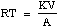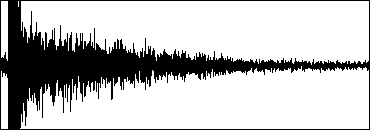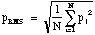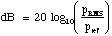Swarthmore College

Engineering Methodology

Reverberation Lab

In lecture this week, you learned about the acoustics of rooms, buildings, and outdoor spaces. The lab assignment below asks you to measure the reverberation time of a space on campus by writing a Matlab script to process a recorded balloon pop.

Problem

The reverberation time (RT) of a room or concert hall is the time it takes for a sound to decay 60 dB from its initial sound pressure level. This quantity can be measured directly or can be expressed via the Sabine formula (1922):(1)

where K is a constant, V is the volume of the hall, and A is the total acoustic absorption of materials in the room: A = SaiSi. To calculate this sum, the acoustic absorption coefficient a of each material in the room is multiplied by the area S which it covers. For V in ft3 and S in ft2, K = 0.049 sec/ft., and for V in m3 and A in m2, K = 0.161 s/m. The a are tabulated from careful tests performed previously on samples of the same material in a very reverberent space (echo chamber). As long as the room whose RT is being measured has acoustic absorption spread nearly uniformly on all surfaces, and as long as the aspect ratio of the hall does not deviate too far from 1:3, Equation 1 gives good agreement with direct measurement. In this lab we will test this assertion.

Form a lab group and go to a location on campus of your choosing. Estimate the volume of the space and the acoustic absorptions of each surface using the coefficients appended here (find the a of the material that is most similar to each surface finish if there is no exact match). Calculate the total absorption and use Equation 1 to estimate the expected RT for the space (sketches, calculations, and commentary should go in your lab notebook). Tape-record a balloon pop in the space and play back the tape into SoundEdit™ to edit the sound and observe the decay. Using SoundHandle™, convert the sound of the pop into an ascii text file that Matlab can open as data.

Write a Matlab script that opens the ascii text file containing the balloon pop sound (use the load command) and then calculates the reverberation time by fitting a best-fit straight line to the decay expressed in dB. Using the drop box on the Classes file server, hand in your script only (with your username followed by the number 9 as the script name before the ".m").

Solution

Using the recording Walkman, find a place on campus whose reverberation time you would like to measure. Record a balloon pop with the microphone located about 10 feet (3 m) from the balloon and then play back the recording while you listen on the headphones, re-setting the counter to zero at the sound of the pop. Take the recorder to a non-PowerPC Mac that can run SoundEdit™ 2.0.5 (in Hicks 314 or equivalent) and connect the recorder to the computer using a patch cord (the stereo jack end of the cord should be in the tape player's headphone plug and one of the mono jack ends of the cord should be in the computer's microphone input plug). Rewind the tape slightly and play back the tape while SoundEdit is recording (hint: best results are obtained when the volume control of the tape player is set quite low but not too low; you may have to rewind and try several different volume control settings). Experiment to get the least clipping of the pop while obtaining a good decay shape such as this:While still in SoundEdit™, trim off the beginning and end of the sound so that the decay begins immediately with the leftmost sample (you may wish to view the frequency and time waveforms and make notes on them in your lab notebook). Save the sound (using "Save As...") in IFF format and quit SoundEdit™. Launch SoundHandle™ and open the balloon pop as an AIFF file but save it again (using "Save As...") as a Text file (the size of this file will be about three times longer than the more compact IFF format). The SoundHandle step is necessary because SoundEdit does not allow direct conversions of sound files into numerical text. Give each member of your lab group a copy of this text file for the Matlab part of the assignment.

Copy your balloon pop text file into the Matlab folder (or User folder on public machines) and open Matlab. Write an script that opens the text file and reads the data into an array (you may have to take the transpose of the array since the file will be read in as a column, not a row, vector). Also, replace the first point with a zero, since SoundHandle uses the first value for storing the length of the array. Have your script display a time-waveform plot of the balloon pop (SoundEdit uses the default sampling rate of 22254 samples per second, so you can create an appropriate time array) and play the sound to the user. The script should then calculate the RT of the space based on the rate of decay of the pop.

To calculate RT, the data in the array will have to be manipulated by your script. Note that since SoundEdit uses only 8 bits to represent each sound sample point, the range extends from -128 to +128 (a total of 28 = 256 discretization steps; CD-quality audio uses 16 bits and audiophiles claim that even this is insufficient). First convert the data into root-mean-squared (RMS) values using the formula(2)

where each point in the original array is pi and N is the number of points to the right of the current point to be included in the integration (or smoothing) of the decay function (IT IS NOT THE TOTAL NUMBER OF POINTS IN THE ARRAY!). Your program will therefore need to march down the original array, calculating the RMS value of each point based on the N points that follow it. The last N points should be handled as a special case (use an if statement), since otherwise the program would march off the end of the array (I suggest assigning the RMS values to be the same for the last N points). For debugging purposes, you may wish to try several different values of N (N=1000 worked for me) and plot versus time the RMS array that results. Adjust N so that the curve is somewhat smooth but still maintains the shape of the original balloon pop decay envelope (this takes a lot of time to run because the arrays are so large).

Once you have obtained a good smoothing of the RMS data, create a new dB array from the RMS array via the formula(3)

where pref is the maximum value of the RMS array, since in this case we care only about changes relative to the initial (loudest) part of the sound.

Next, calculate the slope of the balloon pop decay in dB/sec by using the best-fit straight line through the data in the dB array (use the polyfit function to fit a first-order polynomial). -60 dB divided by this slope will give the reverberation time in (positive) seconds, which you should print out to the user.

Challenge assignment (only if you find the assignment above unchallenging)

Have the program filter the sound (using the butter function) in both high- or low-pass before the best-fit slope calculation to provide the user with an idea of the variation of reverberation time with frequency in the space.